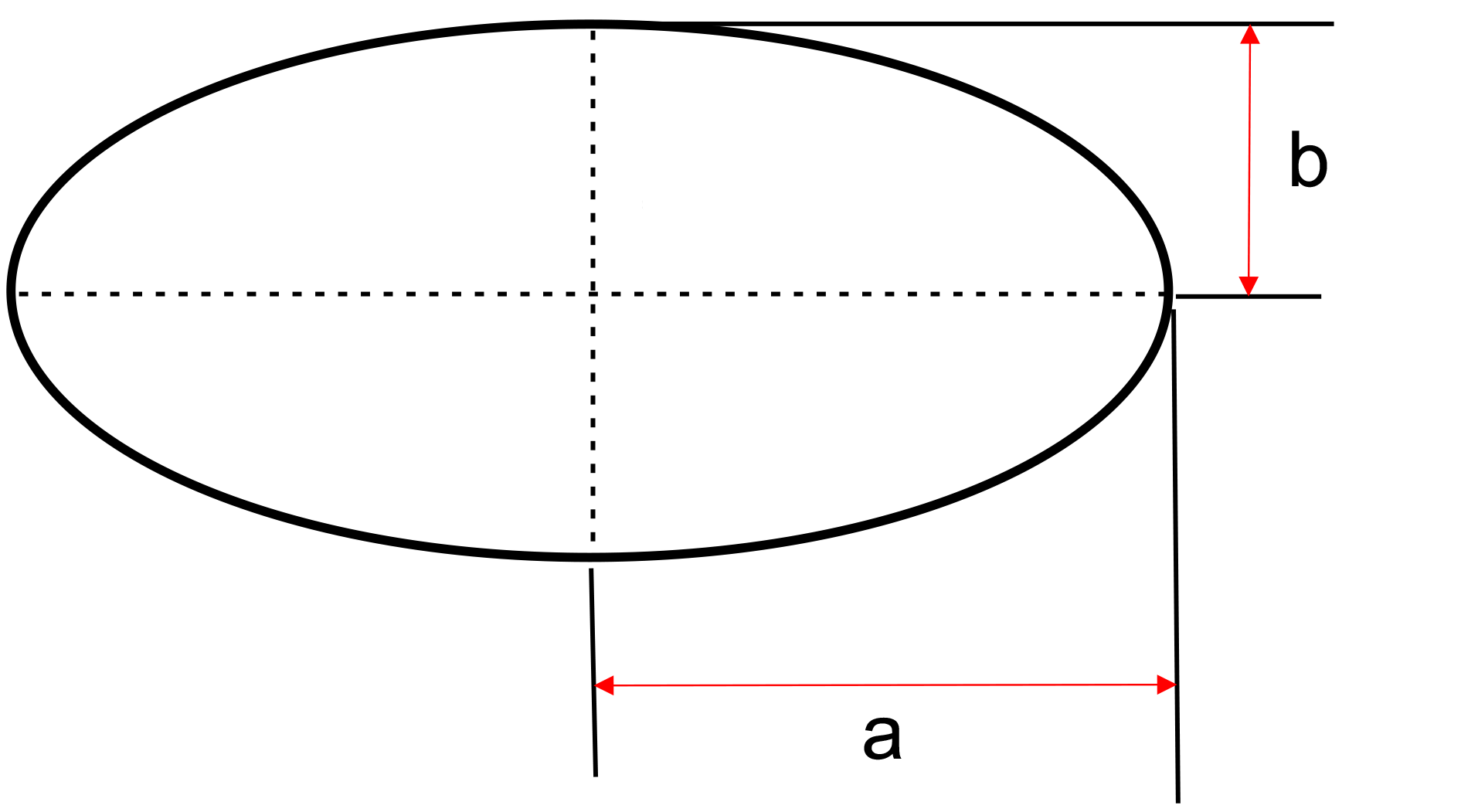﻿ Ellipse: - on line calculation, formula - FORMIAX

# Ellipse - circumference and surface areaC ≈ π × √2 × (a2 + b2)

S = π × a × b

C = circumference

S = surface area

a = long half axis

b = short half axis

WHAT IS IT ?
Ellipse is defined by two points, each called a focus. If you take any point on the ellipse, the sum of the distances to the focus points is constant.

CALCULATION:

Enter unit e.g.: inch

Enter long half axis "a"

Enter short half axis "b"

Round to number of decimals places

YOU MIGHT BE INTERESTED: